# 数字图像处理？

## November 19, 2018 • 程序 • 阅读设置

GitHub: Bokjan/LabDIP的Release中可以找到生成好的二进制。

# 突如其来的stress

1. 功能区可以分tab页，按照后续功能添加；
2. 图像显示区域需考虑图像的缩放与自适应显示；
3. 参数输出区，用以显示过程，以及相关统计数据和调试信息，可滚动，可选择，可复制，可清除。
阅读程序框架，继续采用Windows多线程和OpenMP两种方式，补充实现下述功能。算法需自行实现，不能直接使用OpenCV函数。
4. 采用三阶插值的图像任意角度旋转与缩放
5. 图像的傅立叶变换，并与功能1联动，输入图像经过旋转、缩放后的傅立叶变换结果可在右侧显示
6. 给图像添加高斯噪声
7. 采用采用平滑线性滤波、高斯滤波、维纳滤波三种方法过滤不同参数的高斯噪声

# MFC相关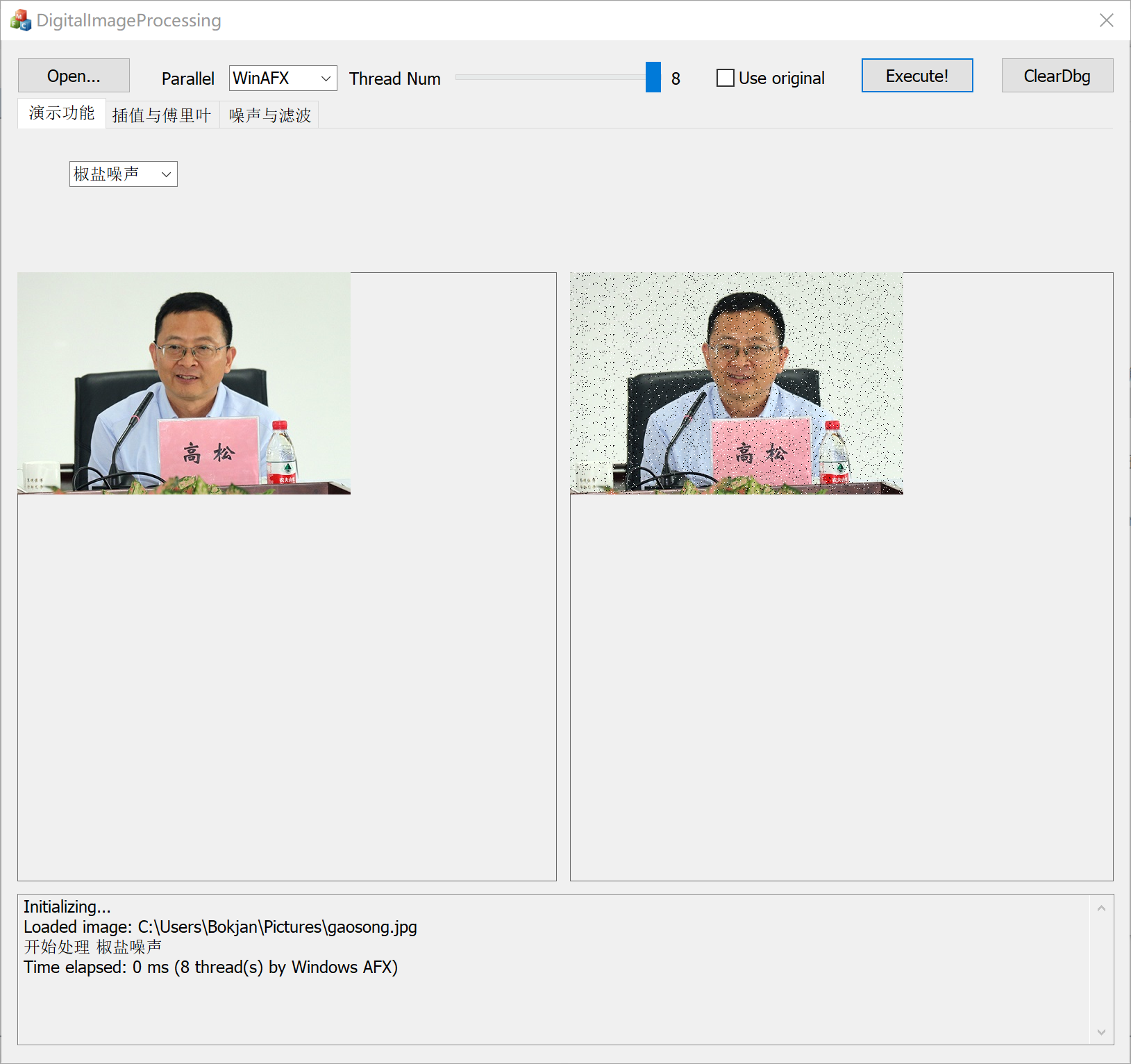## CImage

CImage是ATL/MFC中的一个图像类，可以用它来读取图片并且进行处理。考虑到之后处理图片，写代码的效率和执行效率两个方面，可以考虑将CImage包起来。

class CImageWrapper
{
private:
CImage *img;
public:
byte *mem;
bool IsGray;
int Width, Height, Pitch, BytePP;
inline CImageWrapper(CImage *img) :
img(img), Pitch(img->GetPitch()),
Width(img->GetWidth()), Height(img->GetHeight()),
BytePP(img->GetBPP() / 8), IsGray(BytePP == 1),
mem((byte*)img->GetBits()) { }
inline void SetPixel(int x, int y, const byte value)
{
*(mem + Pitch * y + BytePP * x) = value;
}
inline void SetPixel(int x, int y, const byte r, const byte g, const byte b)
{
*(mem + Pitch * y + BytePP * x + 0) = r;
*(mem + Pitch * y + BytePP * x + 1) = g;
*(mem + Pitch * y + BytePP * x + 2) = b;
}
inline void SetPixel(int x, int y, const byte *ptr)
{
*(mem + Pitch * y + BytePP * x + 0) = ptr;
*(mem + Pitch * y + BytePP * x + 1) = ptr;
*(mem + Pitch * y + BytePP * x + 2) = ptr;
}
inline byte* GetPixel(int x, int y)
{
return (byte*)(mem + Pitch * y + BytePP * x);
}
};

CImage::GetBits得到的是逻辑上一张图片最左上方的像素的起始地址；CImage::GetPitch得到的某处到下一行的同一列的偏移量（每行的长度并不严格等于每一行像素所占的空间，还加了私货）；而CImage::GetBPP则得到的是每个像素所占的二进制位数。其实这个实验有不少保证的，比如都是彩色图像，每个像素均是24比特大小等。那么上面频繁出现的mem + Pitch * y + BytePP * x + N其实就是对应$(x,\ y)$像素$N$通道的内存地址了。多写几个重载呢，使用起来比较简便。

## AFX消息机制下的多线程实现

### 创建一个线程

CWinThread* AFXAPI AfxBeginThread(AFX_THREADPROC pfnThreadProc, LPVOID pParam,
int nPriority = THREAD_PRIORITY_NORMAL, UINT nStackSize = 0,
DWORD dwCreateFlags = 0, LPSECURITY_ATTRIBUTES lpSecurityAttrs = NULL);

typedef UINT (AFX_CDECL *AFX_THREADPROC)(LPVOID);

struct ParallelParams
{
CImage *img;
int wParam;
int begin, end;
void *ctx, *thctx;
void(*cb)(ParallelParams*);
ParallelParams(void) :
wParam(1),
cb(nullptr),
img(nullptr),
ctx(nullptr),
thctx(nullptr) { }
};

### 线程函数的结构

UINT Algo::SaltAndPepperNoise(LPVOID _params)
{
srand(static_cast<unsigned int>(time(nullptr)));
auto params = (ParallelParams*)_params;
CImageWrapper img(params->img);
for (int i = params->begin; i < params->end; ++i)
{
int x = i % img.Width;
int y = i / img.Width;
if ((rand() / (double)RAND_MAX) <= NOISE_FACTOR)
{
byte val = (rand() & 0x1) ? 0 : 255;
img.SetPixel(x, y, val, val, val);
}
}
PostMessageW(DA->HWnd, WM_USER_EXECUTE_FINISHED, params->wParam, (LPARAM)params);
return 0;
}

### 注册消息处理函数

ON_MESSAGE(WM_USER_EXECUTE_FINISHED, &CDigitalImageProcessingDlg::OnExecuteFinished)

### 编写消息处理函数

LRESULT CDigitalImageProcessingDlg::OnExecuteFinished(WPARAM wParam, LPARAM lParam)
{
static int cnt = 0;
++cnt;
if (DisplayAgent::GetInstance()->GetThreadOption().count == cnt || wParam == 0)
{
cnt = 0;
auto p = (ParallelParams*)lParam;
if (p->cb != nullptr)
p->cb(p);
DA->PrintTimeElapsed();
DA->PaintCImageToCStatic(((ParallelParams*)lParam)->img, &mPictureControlRight);
if (p->ctx != nullptr)
delete p->ctx;
if (p->thctx != nullptr)
delete[] p->thctx;
}
this->mButtonExecute.EnableWindow(true);
return LRESULT();
}

# 算法

## 加噪

### 高斯噪声

\begin{aligned} Z_0&=\sqrt{-2\ln{U_1}}\cos(2\pi U_2) \ Z_1&=\sqrt{-2\ln{U_1}}\sin(2\pi U_2) \end{aligned}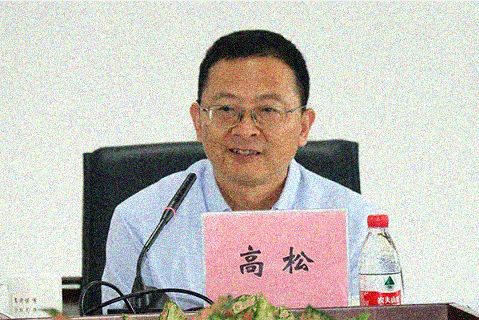## 双三次插值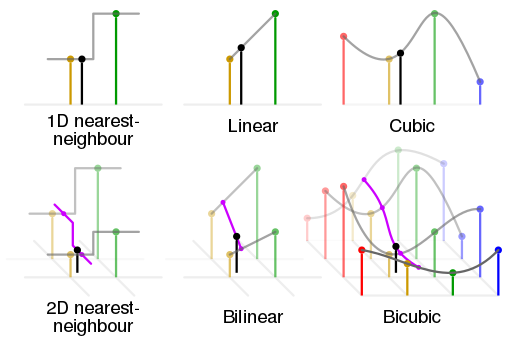W(x)=\left{\begin{matrix} \begin{aligned} &(a+2)|x|^3-(a+3)|x|^2+1 &&\text{for}\ |x|\leq 1, \ &a|x|^3-5a|x|^2+8a|x|-4a &&\text{for}\ 1<|x|<2,\ &0 &&\text{otherwise} \end{aligned} \end{matrix}\right.

## 滤波器

### 中值滤波器### 高斯滤波器

$$G(x,\ y)=\frac{1}{2\pi\sigma^2}\exp{\left (-\frac{x^2+y^2}{2\sigma^2}\right )}$$

static void GetGaussianTemplate(double t, double stddev)
{
const int center = 1; // [[0, 1, 2], [0, 1, 2], [0, 1, 2]], center is (1, 1)
double total = 0;
static const double PI = acos(-1);
for (int i = 0; i < 3; ++i)
{
double xsq = pow(i - center, 2.0);
for (int j = 0; j < 3; ++j)
{
double ysq = pow(j - center, 2.0);
double f = 1 / (2.0 * PI * stddev);
double e = exp(-(xsq + ysq) / (2.0 * stddev * stddev));
t[i][j] = f * e;
total += t[i][j];
}
}
for (int i = 0; i < 3; ++i)
for (int j = 0; j < 3; ++j)
t[i][j] /= total;
}

### 维纳滤波器

$$\mu{i,j} +\frac{\max(0,\ \sigma^2{i,j}-\nu^2)}{\max(\sigma^2{i,j},\ \nu^2)}\cdot(\text{p}{i,j}-\mu_{i,j})$$

## 傅里叶变换

$$F(u,\ v)=\sum{x=0}^{M-1}\sum{y=0}^{N-1}f(x,\ y)\textrm{e}^{-\textrm{j}2\pi(\frac{ux}{M}+\frac{vy}{N})}$$

$$\left | F(u,\ v) \right |=\sqrt{R^2(u,\ v)+I^2(u,\ v)}$$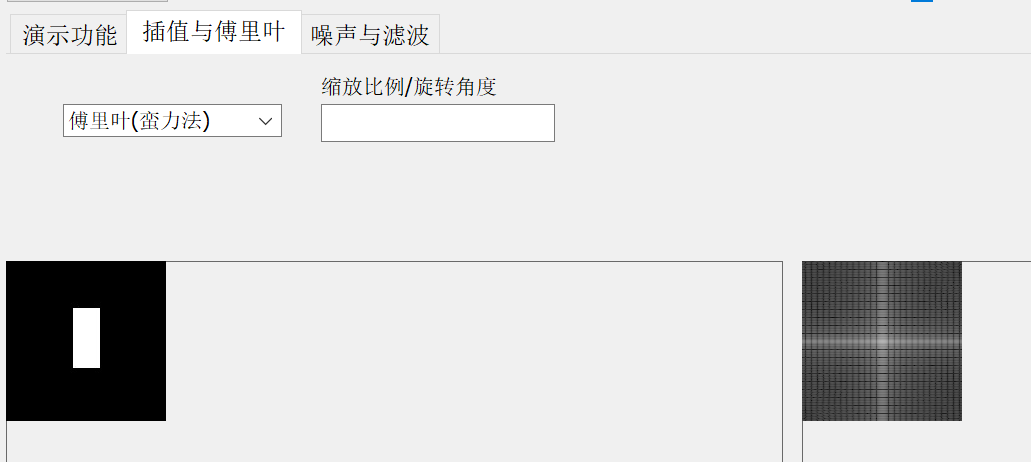# 基于OpenMP的并行化处理

for (int i = 0; i < th.count; ++i)
{
}

#pragma omp parallel for num_threads(th.count)
for (int i = 0; i < th.count; ++i)
{
}

# GPGPU?

## OpenCL Wrapper

class CLAgent
{
private:
static CLAgent clAgent;
CLAgent(void);
~CLAgent(void);

cl_device_id*       Devices;
cl_context          Context;
cl_kernel           Kernel;
cl_program          Program;
cl_command_queue    Queue;
public:
struct Str
{
char* s;
size_t l;
inline void Release(void)
{
delete[] this->s;
}
};
inline static CLAgent* GetInstance(void)
{
return &CLAgent::clAgent;
}
bool LoadKernel(const char *fn, const char * kernel_name);
cl_mem CreateMemoryBuffer(size_t size, void *pointer);
bool ReadBuffer(cl_mem obj, size_t size, void *dst);
bool SetKernelArg(cl_uint index, size_t size, const void *pointer);
bool RunKernel(cl_uint work_dim, const size_t *local_work_size, const size_t *global_work_size);
void Cleanup(void);
};

## 优化

for (int y = 0; y < height; y++)
{
for (int x = 0; x < width; x++)
{
pixelR[index] = *(pRealData + pit * y + x * bitCount + 2);
pixelG[index] = *(pRealData + pit * y + x * bitCount + 1);
pixelB[index] = *(pRealData + pit * y + x * bitCount);
index++;
}
}
MedianFilter_CL(pixelR, pixelIndex, width, height);
MedianFilter_CL(pixelG, pixelIndex, width, height);
MedianFilter_CL(pixelB, pixelIndex, width, height);
index = 0;
for (int y = 0; y < height; y++)
{
for (int x = 0; x < width; x++)
{
*(pRealData + pit*y + x*bitCount + 2) = pixelR[index];
*(pRealData + pit*y + x*bitCount + 1) = pixelG[index];
*(pRealData + pit*y + x*bitCount) = pixelB[index];
index++;
}
}

## 改写的注意事项

### TDR修改

Timeout Detection and Recovery是Windows上处理显卡未响应的功能。如果Windows在一定时间（默认值2s）内没有收到显卡的响应，系统将会重置显卡驱动。因此在进行蛮力傅里叶等非常耗时的操作时，很容易会触发TDR使显卡驱动重启，那么结果就有问题了。我们可以将超时时间设的更长来规避这个问题。在注册表编辑器中找到HKEY_LOCAL_MACHINE\SYSTEM\CurrentControlSet\Control\GraphicsDrivers并添加DWORD项TdrDelay设为一个你觉得合适的值即可。

# 性能对比

CPU 为 Intel i7-4870HQ（4 核 8 线程），GPU 为 AMD Radeon R9 M370X。时间单位为毫秒，计时精度约为 16.67ms。旋转/缩放操作使用的图片尺寸为 2880×1800 像素；高斯滤波器使用图片尺寸为 14400×9000 像素；高斯噪声生成使用图片尺寸为 5760×3600 像素。傅里叶变换使用图片尺寸为 480×320 像素。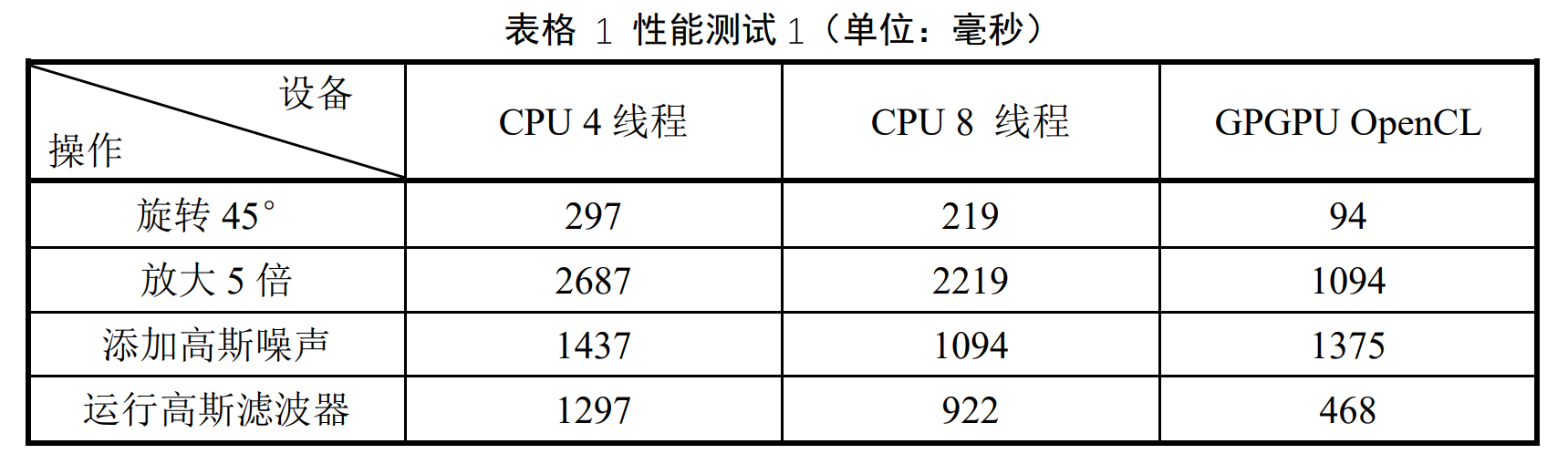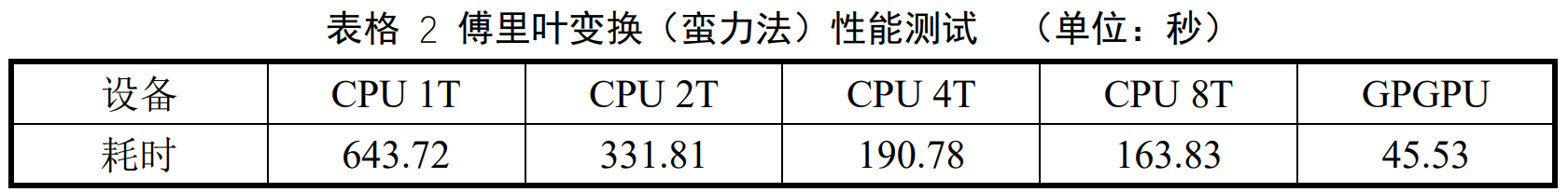# 后记